Courses

# Test: Basic Trigonometric Formula

## 15 Questions MCQ Test Mathematics (Maths) Class 11 | Test: Basic Trigonometric Formula

Description
This mock test of Test: Basic Trigonometric Formula for JEE helps you for every JEE entrance exam. This contains 15 Multiple Choice Questions for JEE Test: Basic Trigonometric Formula (mcq) to study with solutions a complete question bank. The solved questions answers in this Test: Basic Trigonometric Formula quiz give you a good mix of easy questions and tough questions. JEE students definitely take this Test: Basic Trigonometric Formula exercise for a better result in the exam. You can find other Test: Basic Trigonometric Formula extra questions, long questions & short questions for JEE on EduRev as well by searching above.
QUESTION: 1

### cos 68° cos 8° + sin 68° sin 8° = ?

Solution:

Cos68°cos8°+sin68°sin8°
We know,
cosAcosB+sinAsinB=cos(A-B)
Cos(68-8)
Cos60°
=1/2

QUESTION: 2

### cos130° cos40°+ sin130° sin40° is

Solution:

cos(a-b) = Cos a Cos b + Sin a Sin b

Cos(130-40)°=cos90°=0

QUESTION: 3

### Which of the following is correct:

Solution:

sin(x+y) = sinxcosy + cosxsiny
It is the formula

QUESTION: 4

The value of Cos75°is equal to

Solution: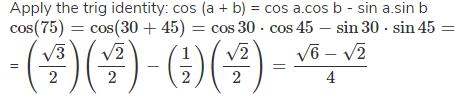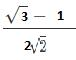QUESTION: 5

Sin A = 1/√10 , Sin B = 1/√5 If A and B are both acute angles, then, A+B =?

Solution:

Solution:- We know that,
sin theta = Opposite/Hypotenuse
therefore, SinA = 1/root10
CosA= 3/root10
similarly, SinB = 1/root5
CosB= 2/root5
now, multiply cos in A+B
Cs(A+B)= CosA x CosB - SinA x SinB substituting the value in above equation we get,
↔3/root10 x 2/root5 - 1/root 10 x 1/root 5
I.e 6/root50 - 1/root50
6-1/5root2. ........(root50=5root2)
5/5root21/ root 2
we know that, sin 45 = 1/ root 2 therefore sin theta/ Cos theta= 45

QUESTION: 6

The value of sin 120° cos 60° + cos 120° sin 60° is equal to

Solution:

Sin A Cos B + Cos A SinB =Sin( A + B)

= Sin(120+60)°  = Sin (180)° = 0

QUESTION: 7

The values of sin 105° is equal to

Solution:

Solution :- sin (105)° = sin (15 + 90) = cos 15°.
First find (cos 15°). Call cos 15° = cos x
Apply the trig identity:
cos2x = 2cos2 x−1
cos2 x = cos (30)° = √3/2 = 2cos2 x−1
2cos2 x = 1 + sqrt3/2 = (2 + sqrt3)/2
cos2 x = (2 + sqrt3)/4
cos x = cos 15° = (sqrt(2 + sqrt3)/2. (since cos 15° is positive)sin(105)° = cos(15)°

QUESTION: 8

Chose which of the following expressions equals sinA + cosA.

Solution:

SinA+cosA=√2(sinA/√2+cosA/√2)
=√2(sinAcosπ/4+cosAsinπ/4)
=√2sin(A+π/4)
by using sin(A+B) formula

QUESTION: 9

The value of cos45°cos15° + sin 45° sin15° is

Solution: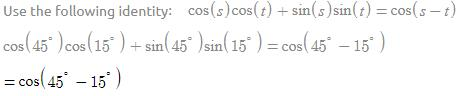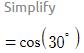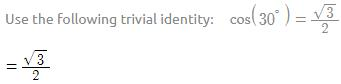QUESTION: 10

sin(60° + A) cos(30° – B) + cos(60° + A) sin(30° – B) is equal to

Solution:

Sin(60+a)cos(30-b)+ cos(60+a)sin(30-b) =sin(60+a+30-b) sin(a)cos(b) + cos(a)sin(b)= sin(a+b) =sin(90+(a-b)) =cos(a-b)

QUESTION: 11

sin(45° – A) cos(45° – B) +  cos(45° – A) sin(45° – B)

Solution:

It is equal to SinACosB + CosASinB = cos(A+B)
hence,
= Sin(45-A+45-B)
= Sin(90-(A+B))
= Cos(A+B)

There's a formula sinAcosB + cosAsinB = sin(A+B) so, here in this question, assume (45-A) as A and (45-B) as B so you'll get sin( 90 -(A+B)) which is cos (A+B)

QUESTION: 12

Sin(n+1)A sin(n+2)A + cos(n+1)A cos(n+2)A =

Solution:

Sin(n+1)ASin(n+2)A + Cos(n+1)ACos(n+2)A =Cos (n+1)ACos(n+2)A + Sin(n+1)ASin(n+2)A =Cos{A(n+2-n-1)} =cos (A.1) =cos A

QUESTION: 13

cos(π/4 - x) cos (π/4 - y) -sin(π/4 - x) sin(π/4 - y) =

Solution:

Cos(π/4-x)cos (π/4-y) - sin (π/4-x) sin(π/4-y)
= CosA*Cos B - Sin A*Sin B
=Cos (A+B)
=cos(π/4-x+π/4-y)
=cos(π/2-x-y)
=cos{π/2 - (x+y)}
= sin(x+y)

QUESTION: 14

cos130° cos40° + sin130° sin40° is equal to

Solution:

cos130°cos40°+sin130°sin40° = cos (130-40)° = cos90° =0

QUESTION: 15

Which of the following is correct:

Solution:

(a) cos (A+B)=cos A cos B - sin A sin B, a is incorrect
(d) cos(A-B)=cos A cos B + sin A sin B. {it is a formula)